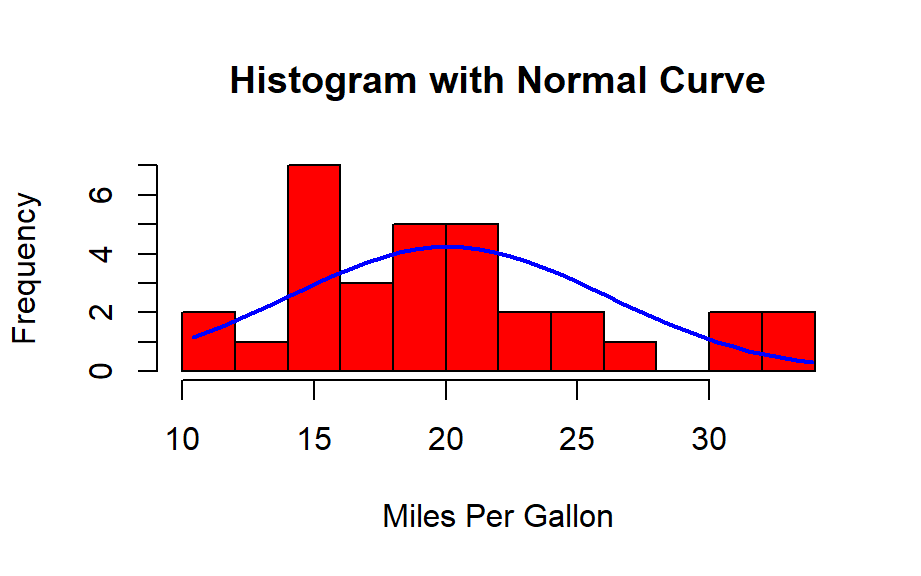August 30, 2017

## Introduction

• This is a quick presentation about some time series plots.

• Really meant as an introduction.

• As a quick way of drawing time series graphs based on ts objects.

• Recall annual, quarterly, monthly ts objects

ts.cy <- ts(rnorm(120,0,1),start = 1900,end = 2020)
ts.qq <- ts(rnorm(120,0,1),start = c(1990,1), frequency = 4)
ts.mm <- ts(rnorm(120,0,1),end = c(2017,8), frequency = 12)
• Lots of resources on the web. Explore and exploit them!

## Sources

There are plenty of internet pages dedicated to illustrating plotting capabilities in R

Also, there is the wonderful package ggplot2 that you should explore at your leisure.

## Simple Time Series Plot

plot(ts(rnorm(120,0,1),1900,2020))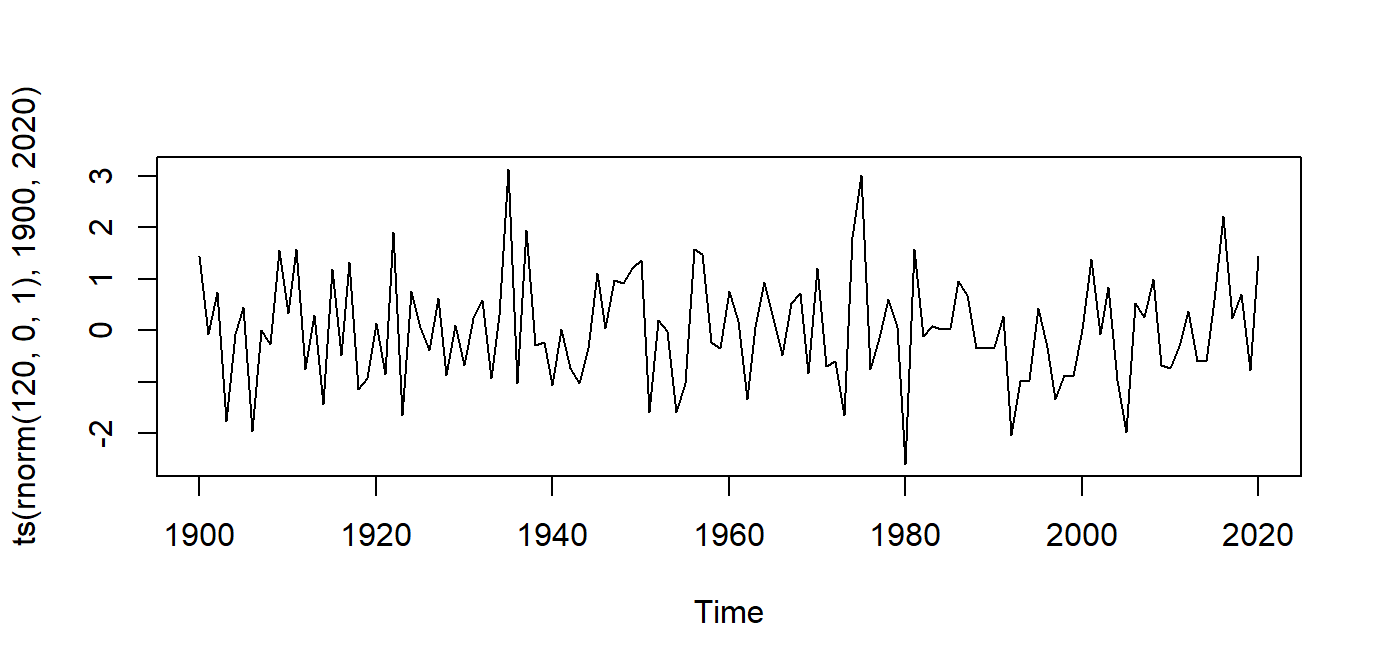## Horizontal y-label

plot(ts(rnorm(120,0,1),1900,2020), las = 1)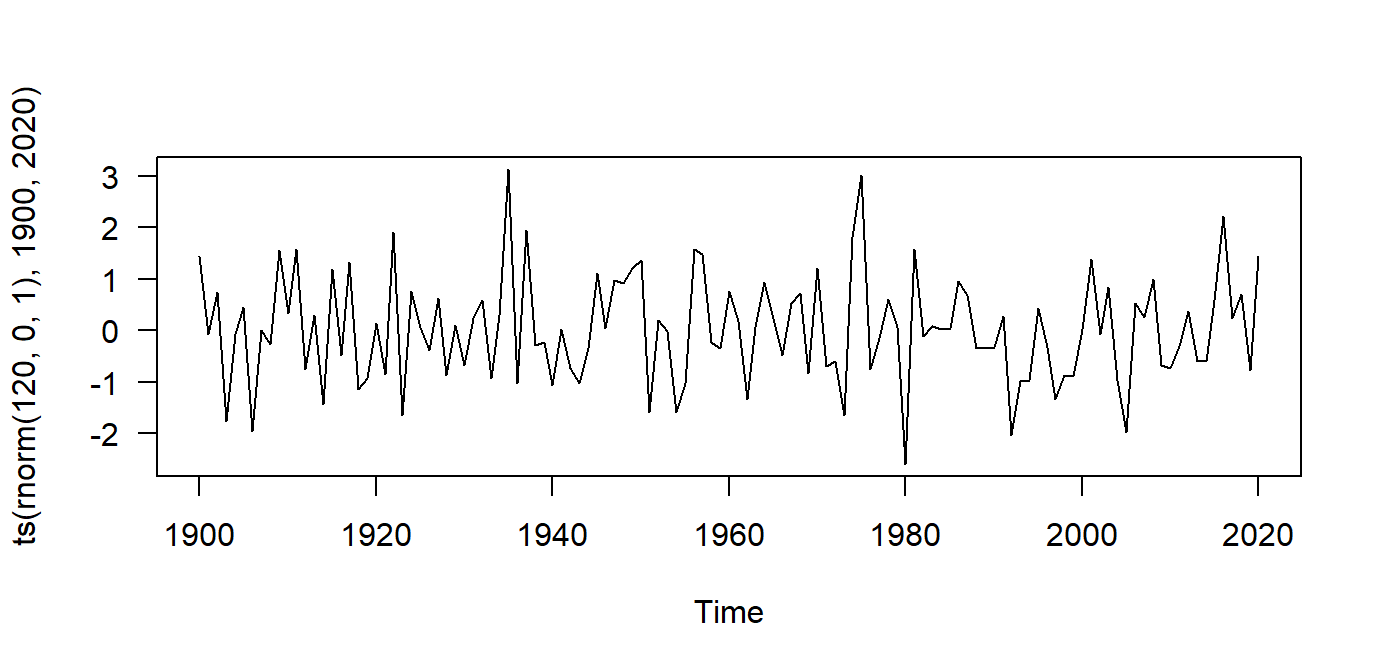## Blue Line Color

plot(ts(rnorm(120,0,1),1900,2020), las = 1, col = "blue")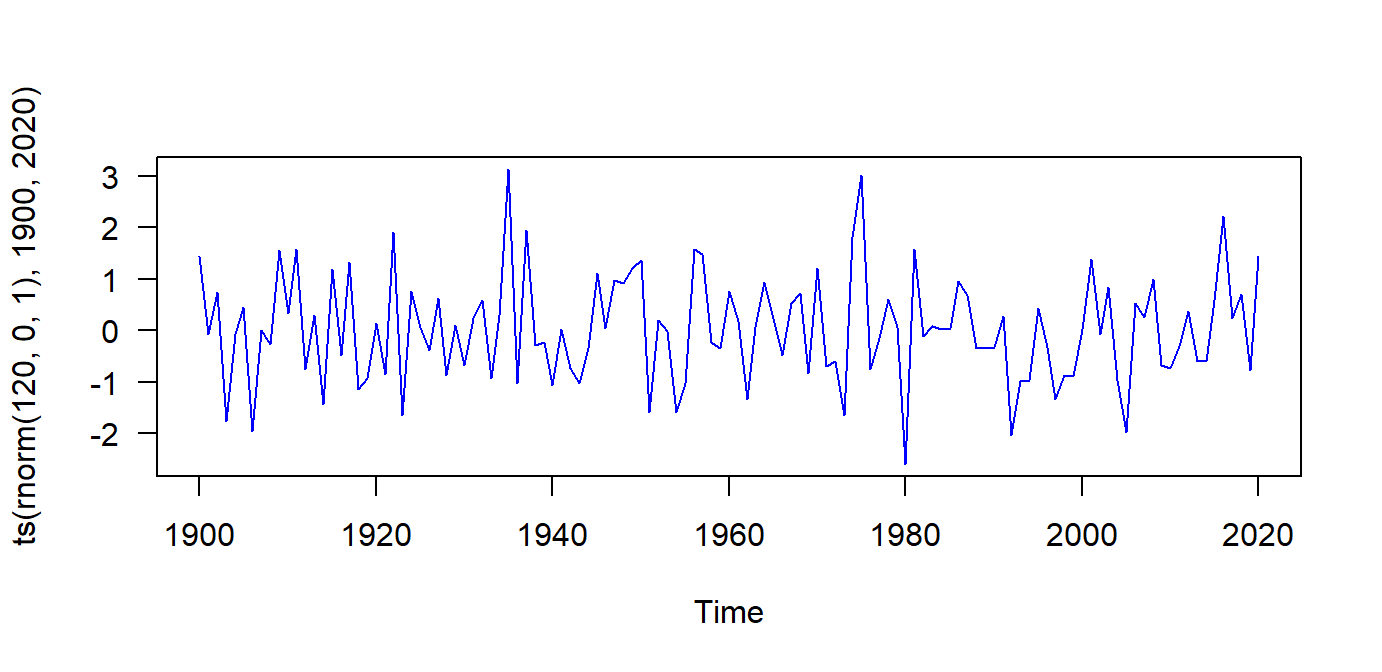plot(ts(rnorm(120,0,1),1900,2020), las = 1, col = "blue", lwd = 3)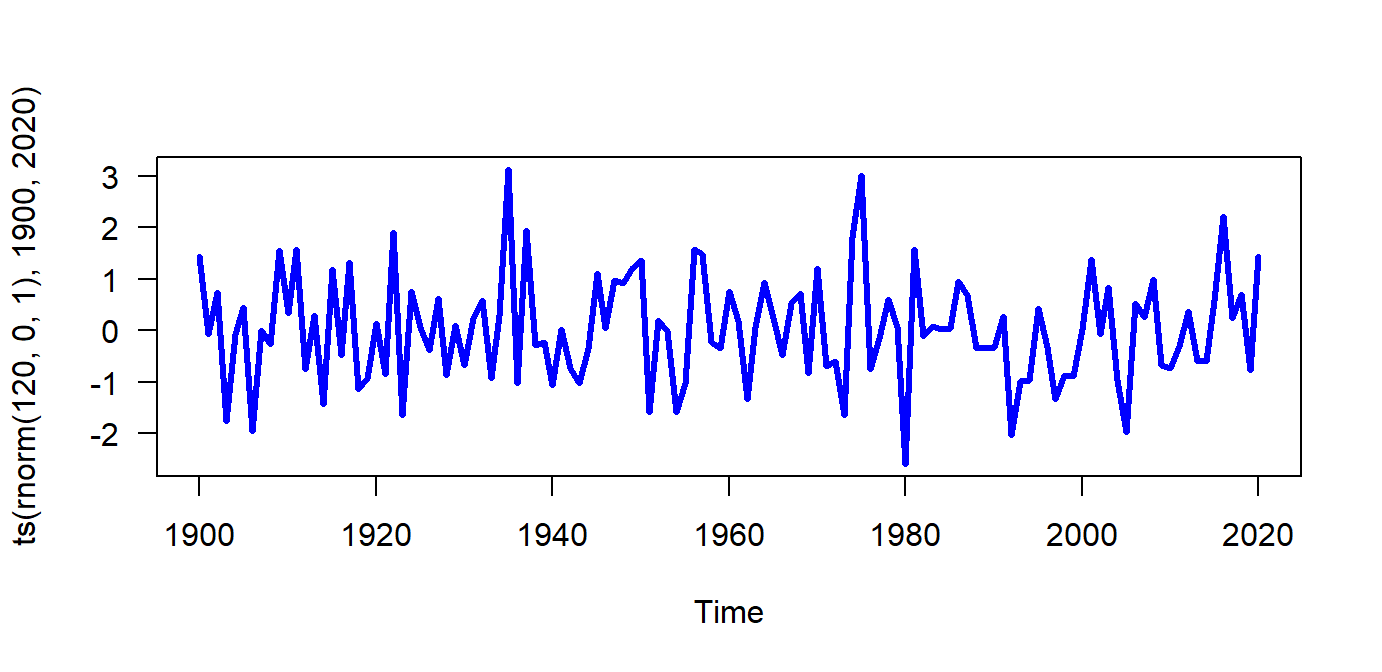## Y-axis Label

plot(ts(rnorm(120,0,1),1900,2020), las = 1,
col = "blue", lwd = 3, ylab = "Realization")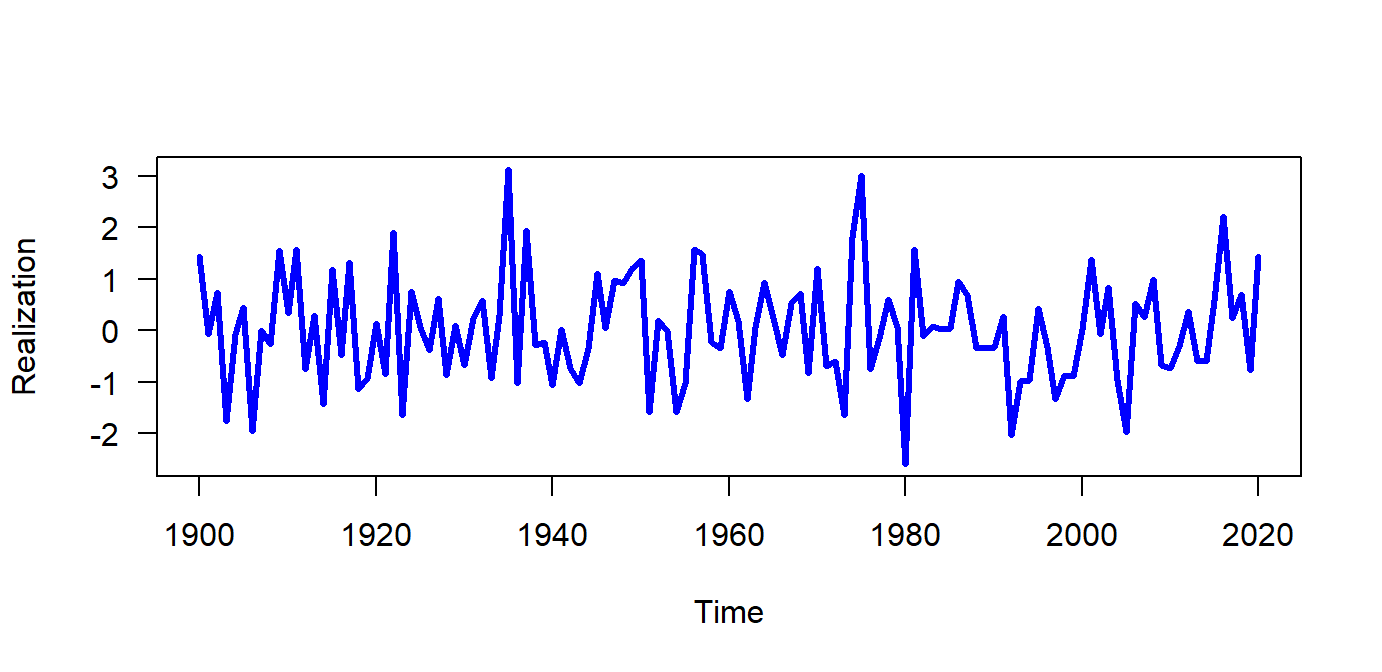abline(h=0)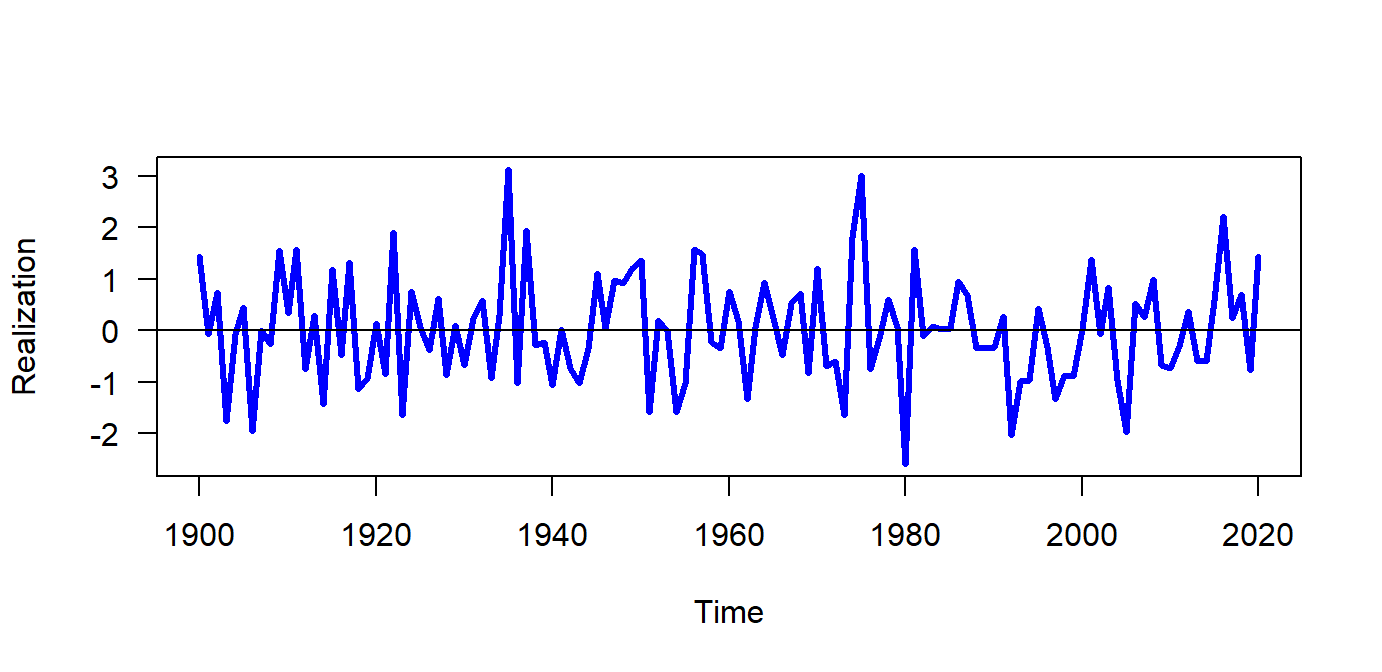## Add a Blue Horizontal Line

abline(h=0, col = "blue")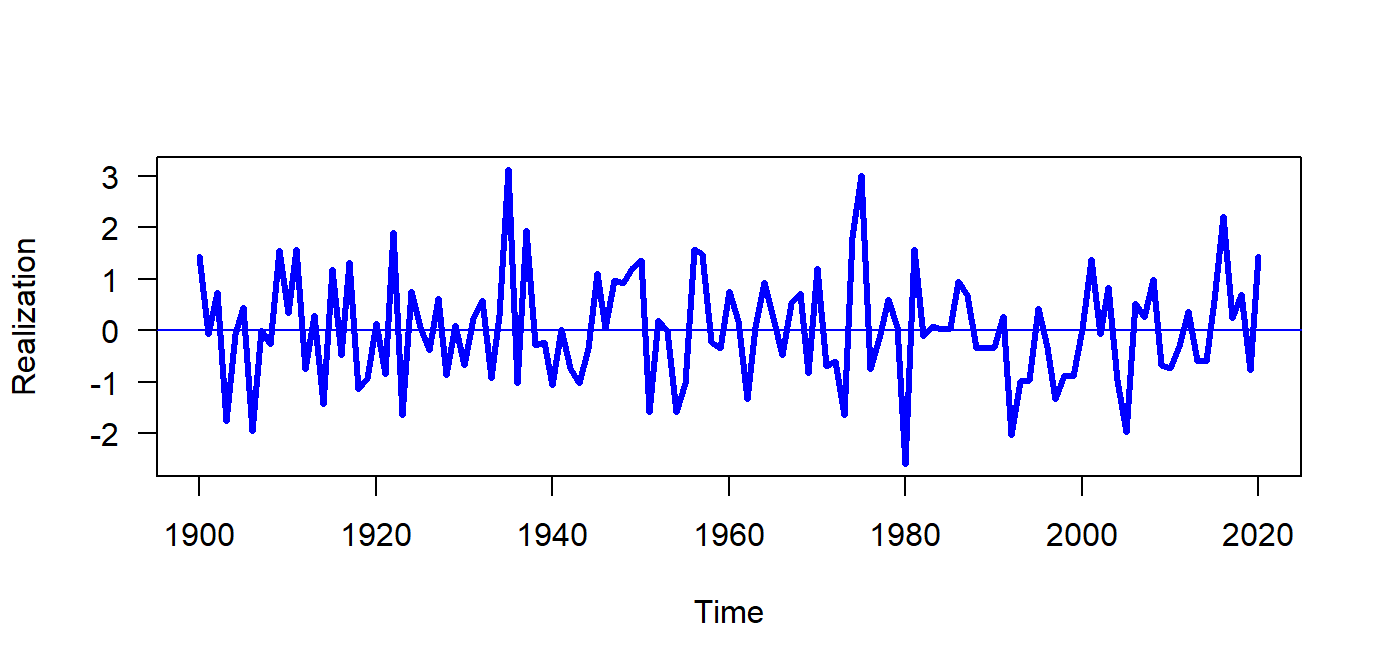## Add a Blue, Thick Horizontal Line

abline(h=0, col = "blue", lwd = 3)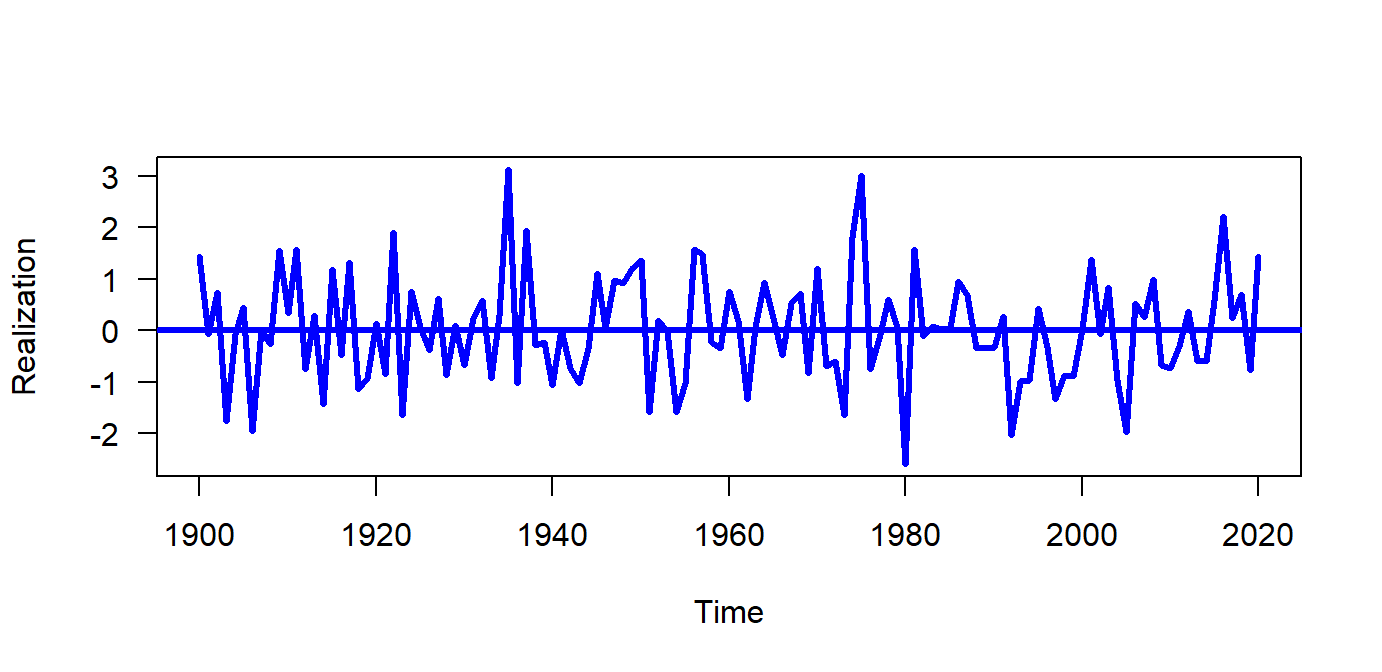## Add Multiple Dashed Horizontal Lines

abline(h=c(0,1,2), lty= "dashed")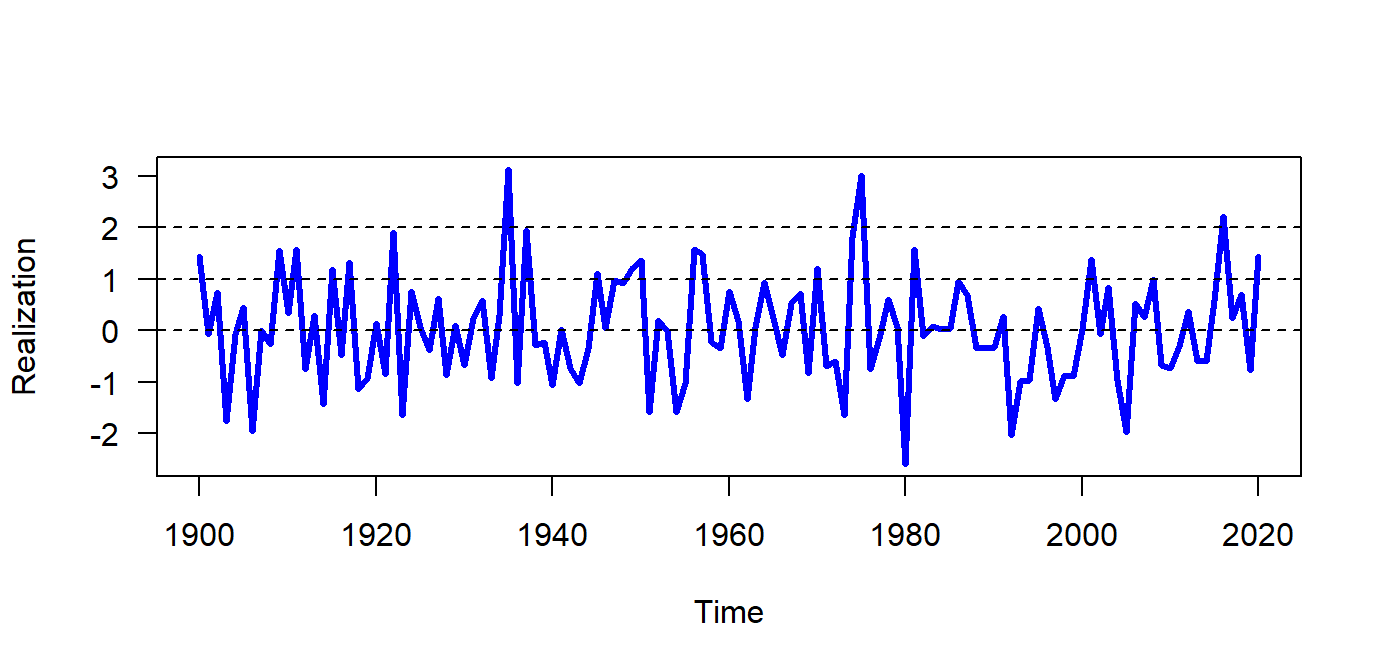## Add a Red Vertical Line

abline(v=2017, col = "red")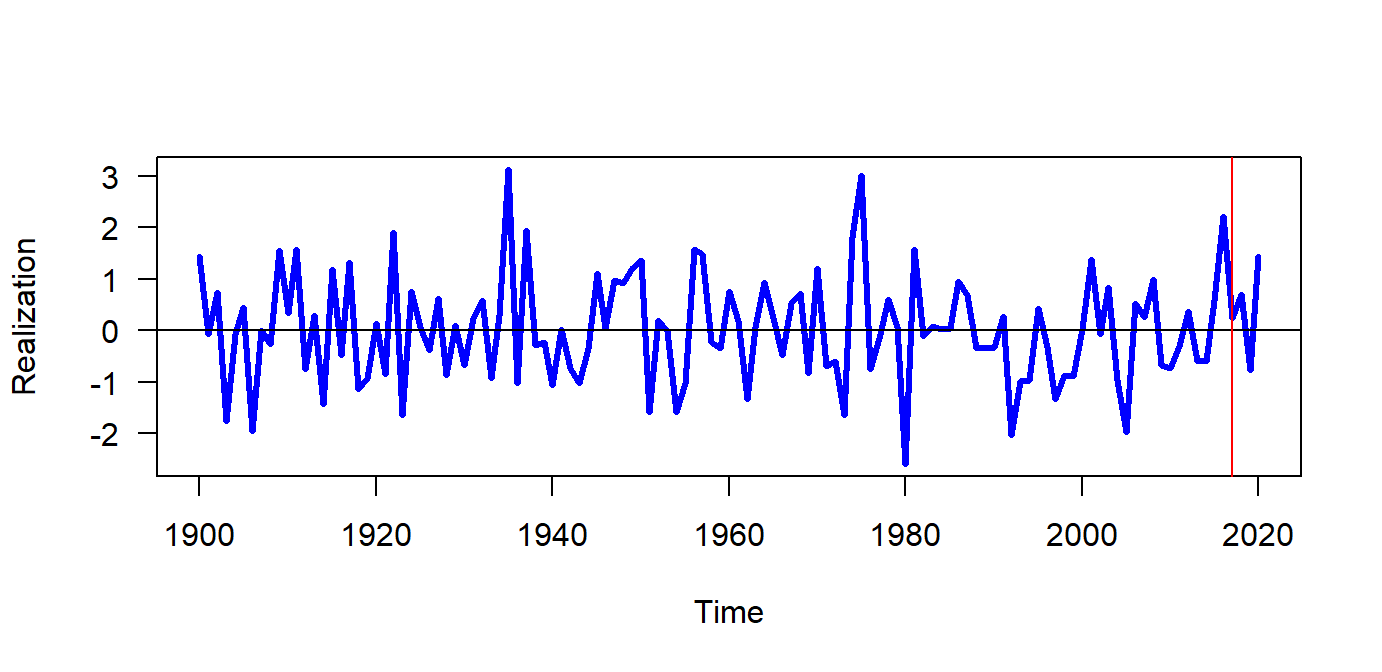## Add Some Text inside the Graph

text(x=2005,y=3,label="This is 2017.",col="red")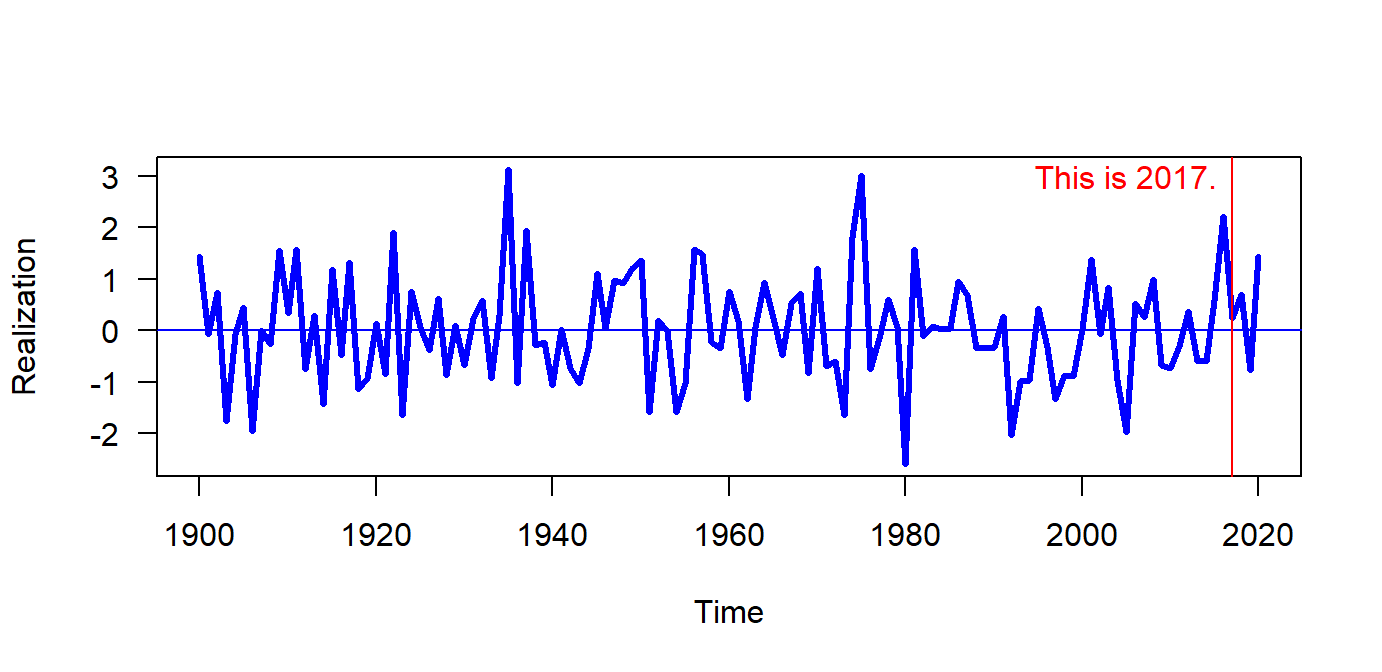abline(v=seq(1900,2020,10), col = "red", lty = "dashed")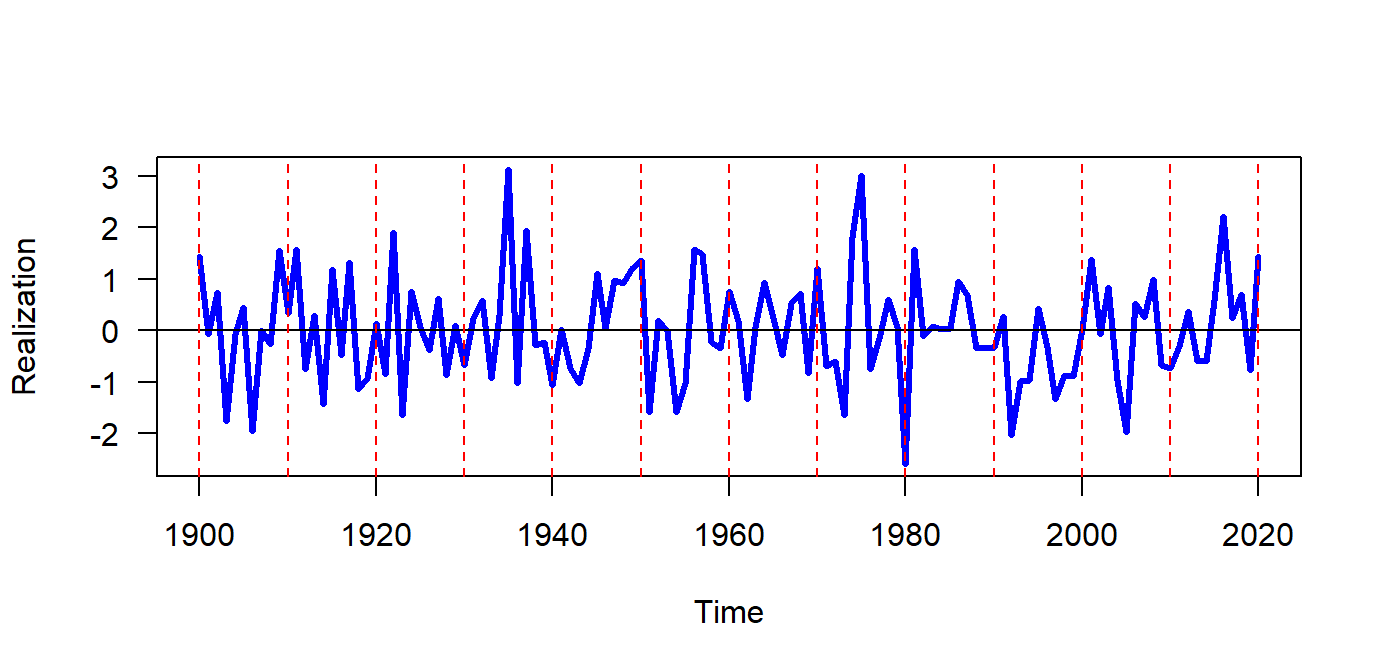## Back to the Simple Starting Graph …

plot(ts(rnorm(120,0,1),1900,2020), las = 1)plot(ts(rnorm(120,0,1),1900,2020), type = "p", las = 1)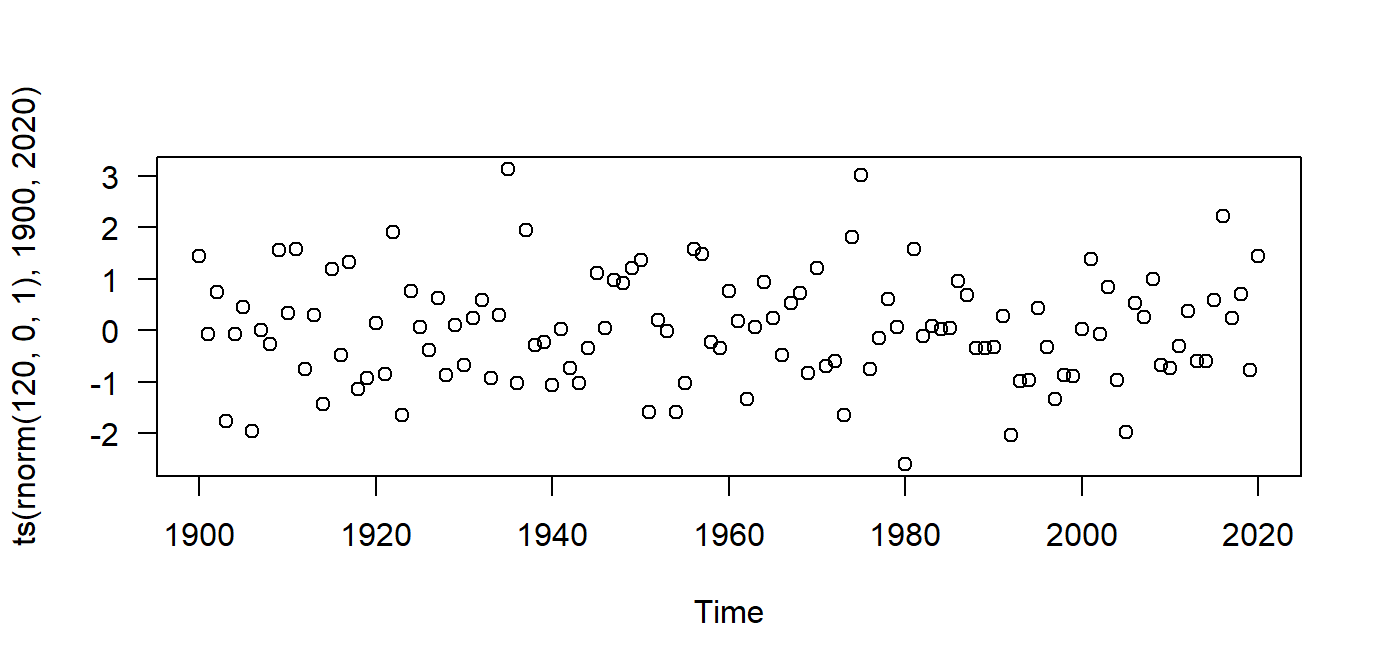## Points and Lines

plot(ts(rnorm(120,0,1),1900,2020), type = "o", las = 1)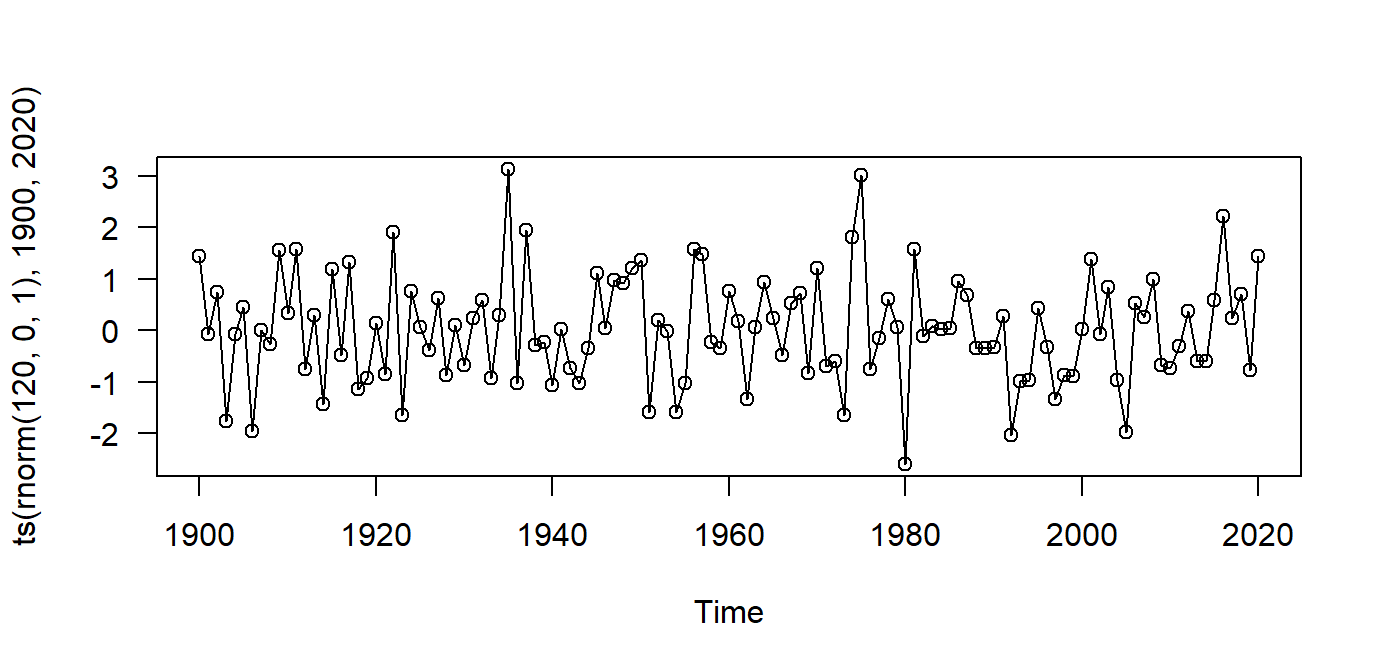## Stair Steps

plot(ts(rnorm(120,0,1),1900,2020), type = "s", las = 1)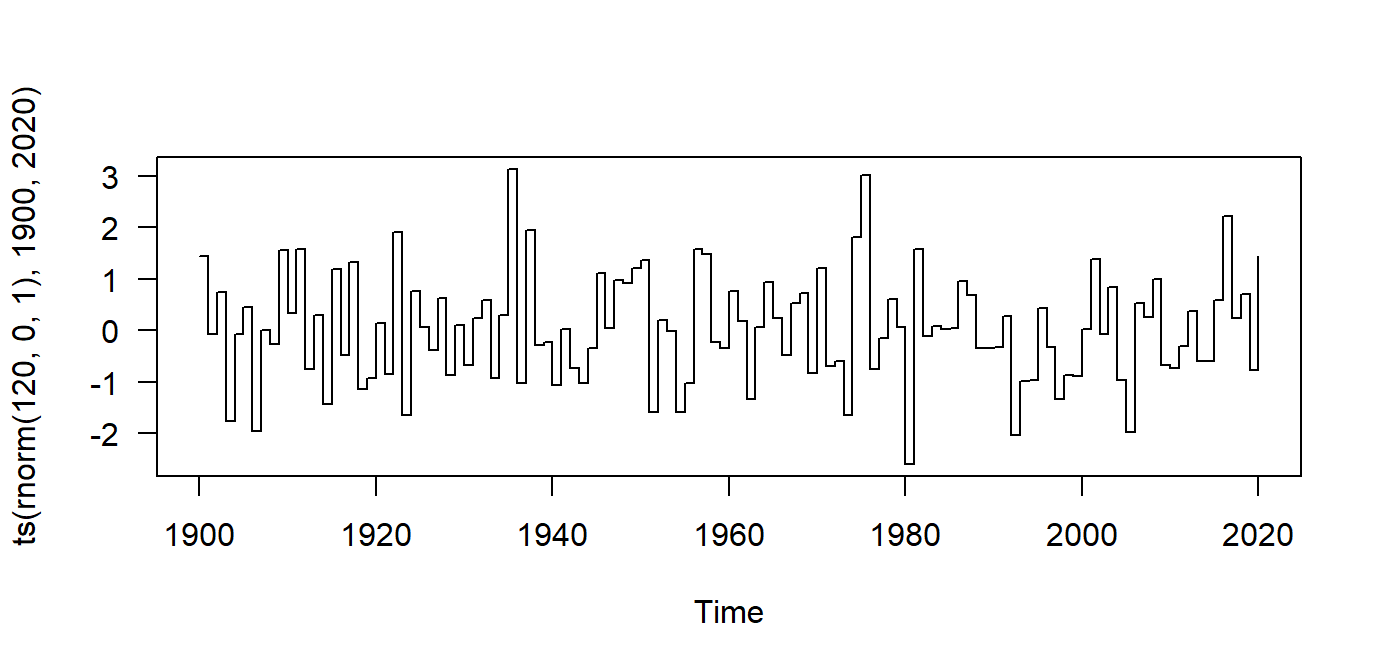## Nothing

plot(ts(rnorm(120,0,1),1900,2020), type = "n", las = 1)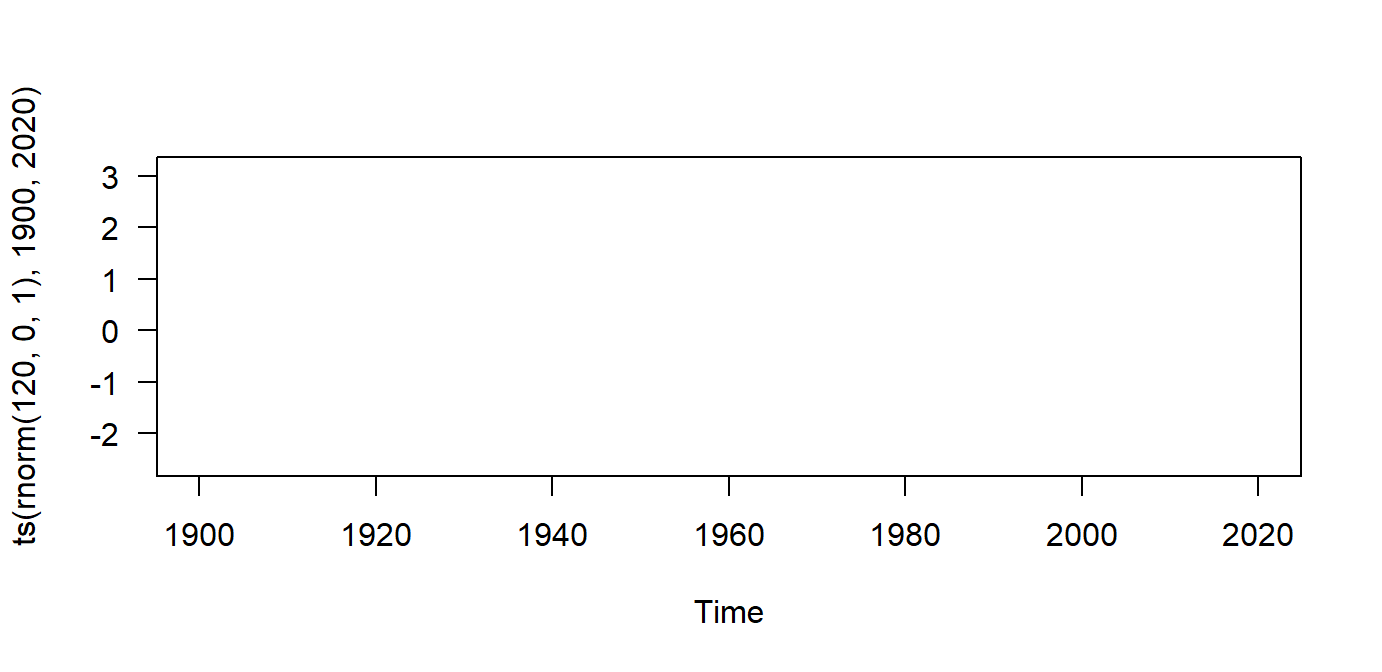## Histogram Like

plot(ts(rnorm(120,0,1),1900,2020), type = "h", las = 1)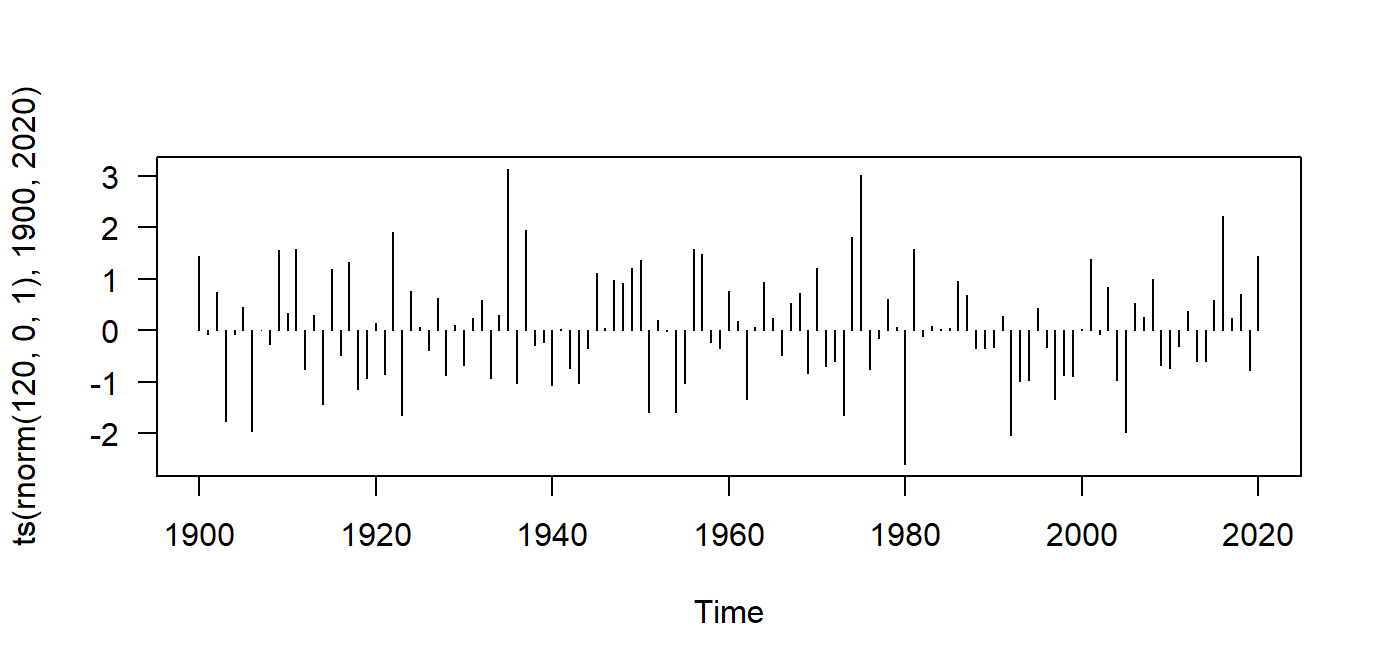## Multiple Time Series

lines(seq(1900,2020,1),ts(rnorm(120,1,1),1900,2020), col = "red")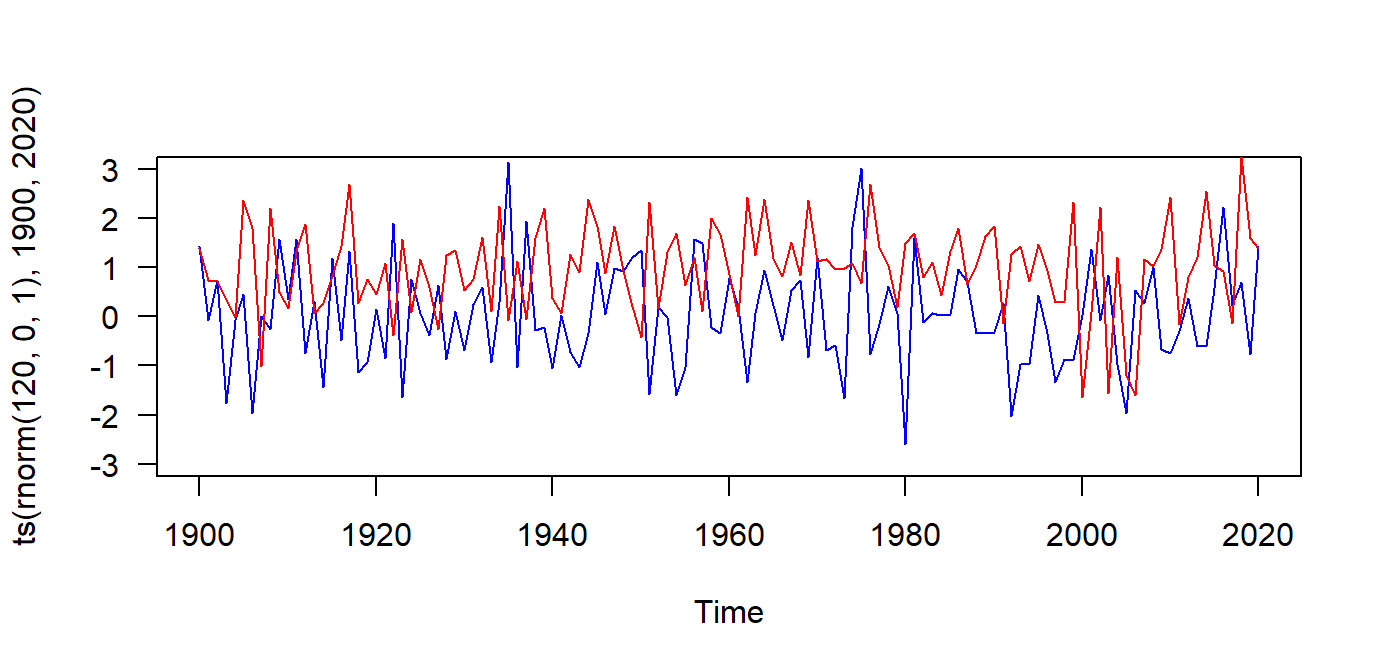legend("bottomleft",col = c("blue","red"),pch = c("-","-"),
ncol = 2, leg.txt <- c("Mean 0", "Mean 1"))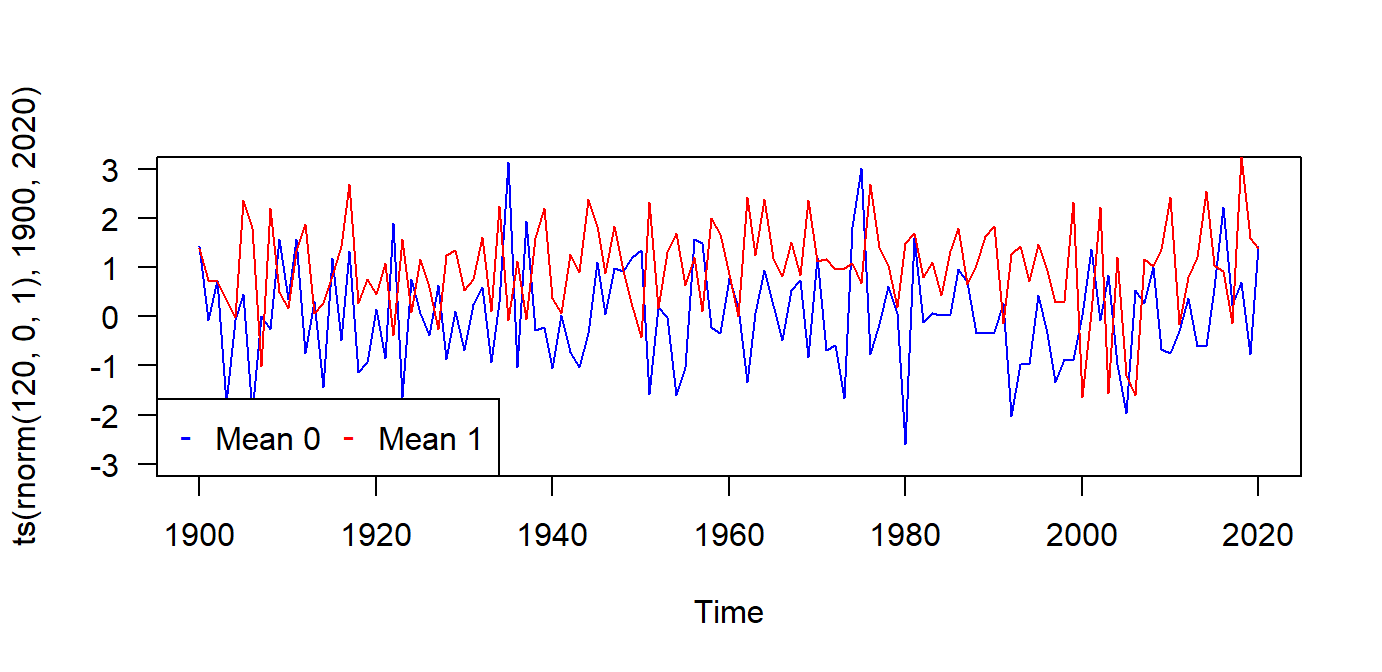## Finding help in R

For example you don't know what which parameter in legend denotes …

?legend

How do I change the color or thickness of a line again?

?lines

hist(mtcars$mpg)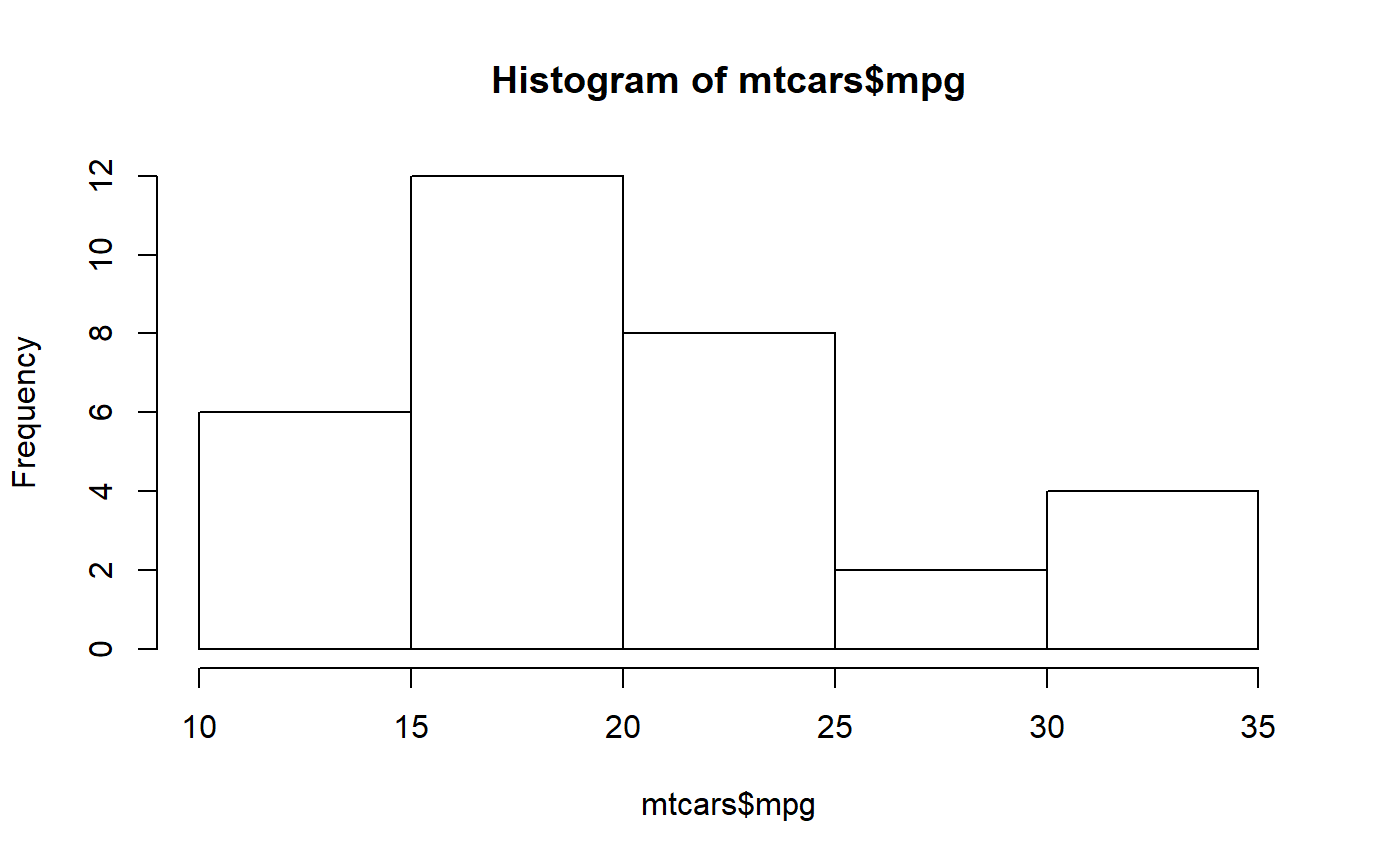## Different Number of Bins and Color hist(mtcars$mpg, breaks=12, col="red")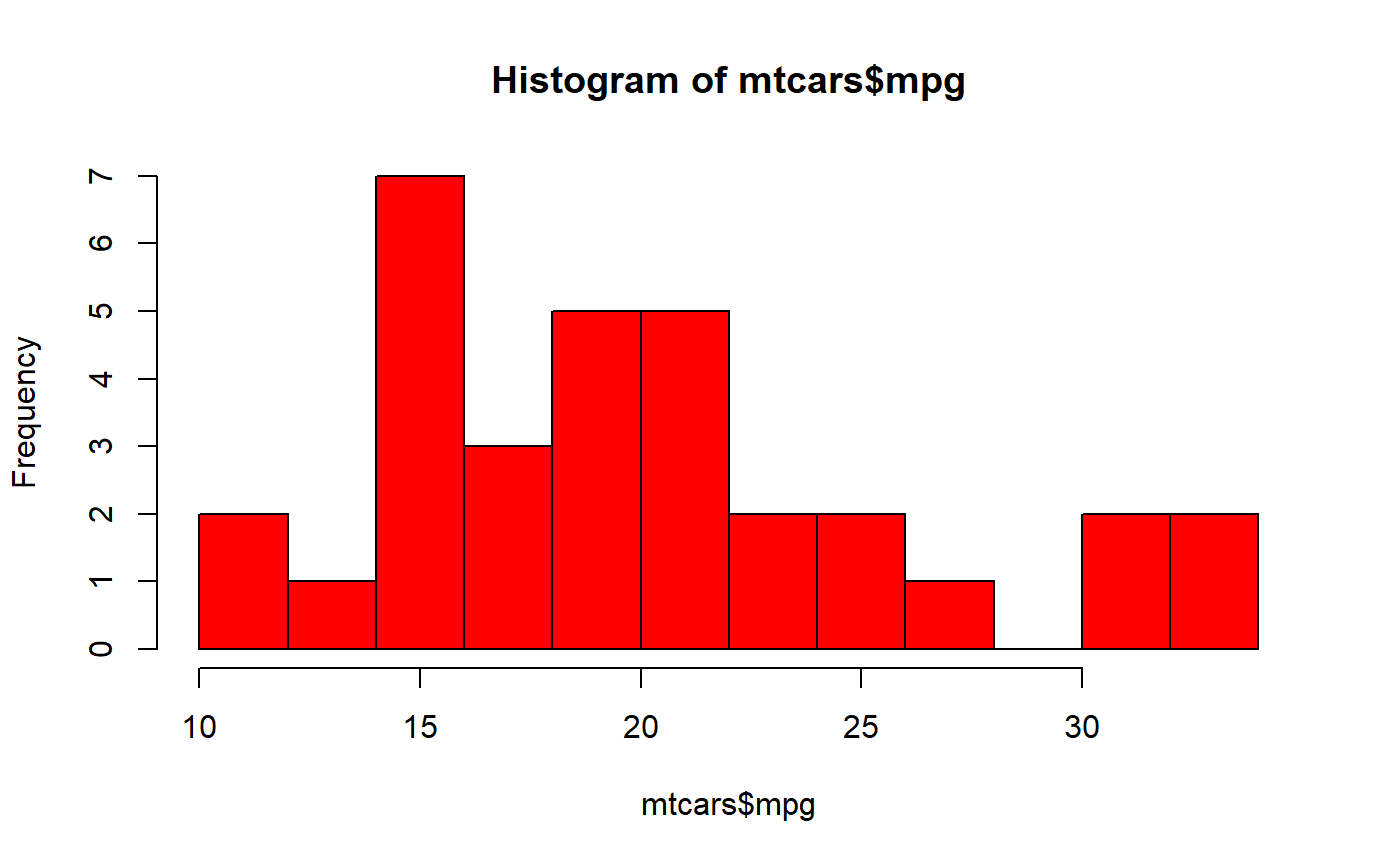x <- mtcars$mpg h <- hist(x, breaks=10, col="red", xlab="Miles Per Gallon", main="Histogram with Normal Curve") xfit <- seq(min(x),max(x),length=40) yfit <- dnorm(xfit,mean=mean(x),sd=sd(x)) yfit <- yfit*diff(h$mids[1:2])*length(x)
lines(xfit, yfit, col="blue", lwd=2)